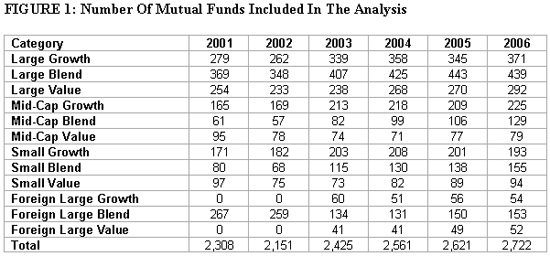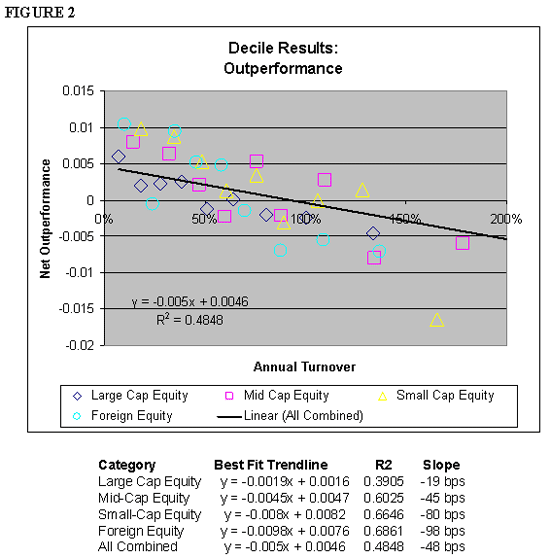# The Pre-Tax Costs Of Portfolio Turnover

May 01, 2007

Outperformance, standard deviation and risk-adjusted performance (defined as the Modified Sharpe Ratio2) were considered. Standard deviation and the Modified Sharpe Ratio were included in order to determine whether or not there was a relationship between turnover and risk; i.e., whether or not higher turnover could be associated with lower risk, as was suggested by Bauman, Miller and Veit (2005). The risk-free rate used for the Modified Sharpe Ratio calculation is defined as the return on the 90-day Treasury bill over the appropriate test period (data obtained from Callan Associates).

The four primary test groups considered for the analysis, along with the subgroups that were reaggregated to determine the total group performance, are listed below:

1. Large Cap Equity: Large Value, Large Blend and Large Growth
2. Mid Cap Equity: Mid Cap Value, Mid Cap Blend and Mid Cap Growth
3. Small Cap Equity: Small Cap Value, Small Cap Blend, Small Cap Growth
4. International Equity: Foreign Large Value, Foreign Large Blend and Foreign Large Growth.3

Since investment style is an important consideration when determining relative outperformance, investments were compared against other investments within the same category and then reaggregated to determine the group performance. For example, all Large Blend funds were tested in one subgroup and all Large Value funds were tested in another subgroup; however, both were combined (on a weighted basis) to determine the aggregate Large Cap equity performance.

The combination weightings are based upon the number funds in the decile for each subgroup. For example, if there were 10 Large Growth fund, five Large Blend funds and five Large Value funds in the Large Cap Equity category, Large Growth would represent 50 percent of the group result for that decile and that period, while Large Blend and Large Value would each represent 25 percent of the total.

The number of mutual funds per asset category that met the previous conditions is included in Figure 1.Although transaction costs differ considerably across foreign markets (see, for example Domowitz, Glen and Madhavan ), for the purposes of this analysis, it is assumed that all foreign equity mutual funds have similar market and country exposures.

Outperformance Results

Figure 2 contains the outperformance for each of the four test groups separated into deciles. The exact figures used for the chart are included in Figure 5. A best-fit linear trend line, considering all groups (i.e., 40 data points), is included to understand the aggregate impact of turnover on outperformance. Below the chart is a table that lists the linear best-fit trend lines, coefficient of determinations (R²) and the slopes of the best-fit trend lines for each of the groups tested.There was a strong relationship between turnover and outperformance for each of the four equity groups tested, with coefficient of determinations (R²) ranging from 0.3905 to 0.6861 (for Large Cap Equity and Foreign Equity, respectively). The slope value represents the cost per 100 percent of turnover for each of the test groups. The cost of turnover increases for smaller market capitalizations (as could be expected), and was largest for foreign equity. The difference between Large Cap Equity and Small Cap Equity (61 basis points) is likely a reflection of the increased liquidity for large capitalization securities.